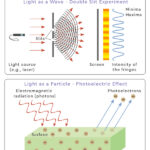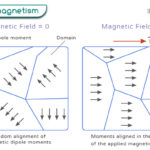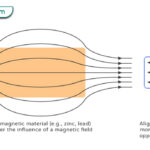Home / Physics / Magnetic Field

# Magnetic Field

## What is Magnetic Field

A magnetic field is an invisible force field generated by a magnet (like bar magnet and horseshoe magnet), moving electric charge (like current-carrying wire, toroid, and solenoid), spinning electrons, and changing electric field. The force due to a magnetic field is called magnetic force. This force can be detected in the presence of magnetic substances. The magnetic field can exist in the air or vacuum.

## What Does a Magnetic Field Look Like

A magnetic field can be pictorially represented by lines typically drawn around either a magnet or a magnetized object. These lines are known as magnetic field lines or magnetic lines of forces. As the magnetic field is a vector quantity, it has both magnitude and direction. The number of lines passing through a given surface is called the magnetic flux. The flux per unit area perpendicular to the direction of the lines is called the magnetic flux density, which gives the strength or magnitude of the magnetic field. A line drawn tangentially at any point on the field lines gives the magnetic field direction. The magnetic field lines are analogous to electric field lines.

### Field Lines in a Bar Magnet

A bar magnet is the simplest form of a magnet that is rectangular. It has two poles, called the north pole and the south pole. The magnetic field lines come out of the north pole and terminate into the south pole. Inside the magnet, they travel from south to north pole. The magnetic field lines can be sketched by bringing a compass near to the magnet. The compass aligns along these lines, and the needle points toward the direction of the magnetic force.

When two bar magnets are brought closer, the magnetic field will be distorted. In this case, opposite poles attract and similar poles repel. The field lines are indicated in the image below.

### Earth’s Magnetic Field

Earth has a magnetic field of its own, which protects life from solar radiation. Earth’s magnetism can be imagined as a giant bar magnet tilted at an angle of 11 degrees to its axis and having two poles. The field lines go out of Earth near the South Pole, enter Earth in the North Pole, and are not aligned with the geographic poles. Earth’s magnetic field is due to currents in the molten materials making up the core, which rotates along with the Earth.

## How to Create Magnetic Field

As mentioned before, a magnetic field is produced when electric charges are in motion. There are two fundamental ways to create it.

### 1. Magnetic Field Due to a Current Through a Straight Conductor

When charges are in motion, current flows through a conductor. The current is defined as a charge flowing per unit time. According to French physicist and mathematician André-Marie Ampère, moving charges in a conductor produce a magnetic field.

Magnetic Field Equation

The magnetic field can be calculated using Ampere’s law. According to this law, the magnetic field produced by an electric current is directly proportional to the current and inversely proportional to the distance from the conductor. Therefore, the formula for the magnetic field’s magnitude due to a current-carrying conducting wire is given by,

B = μoI/2πr

Where,

B: Magnetic field

I: Current

r: Distance from the conductor

μo: Permeability of free space (= 4π x 10-7 N.s2/C2)

SI Unit: Tesla

CGS Unit: Gauss

Dimension: [M1 L0 T-2 I-1]

Direction of Magnetic Field

The right-hand rule gives the direction of the magnetic field. According to this rule, if the thumb indicates the current’s direction, the fingers curling around the conductor indicate the magnetic field’s direction.

### 2. Motion of Electrons Around the Nucleus of an Atom

Electrons move around the nucleus of an atom because of the strong electrostatic force with the protons. The electrons also spin around their axis. Their motion generates an electric current causing the electron to act like a microscopic magnet, thus producing microscopic magnetic fields. The movement of electrons is critical in determining the magnetic nature of some specific materials.

Types of Magnets Based on Electron Spin

If all the electrons spin in the same direction, their spins add up to form a magnetic field, which can be felt on a macroscopic scale. Such kind of behavior is seen in ferromagnets like iron, nickel, and cobalt. These materials make permanent magnets, like bar magnets, and have strong magnetic fields.

On the other hand, if the spins are in opposite directions such that they cancel each other out, the material will not show any magnetism. However, when an external magnetic field is applied, the electron spins are aligned parallel to the field. In such a case, the material displays magnetic behavior. It continues to remain so as long as the field is applied. This type of behavior is seen in paramagnets. A few examples of paramagnets are aluminum, calcium, and oxygen.

## Magnetic Flux vs. Magnetic Field

The following table gives the difference between magnetic flux and magnetic field.

## FAQs

Q.1 What does a changing magnetic field induce?

Ans. A changing magnetic field induces a current.

Q.2. How to measure magnetic field?

Ans. The magnetic field strength can be measured using a magnetometer

Q.3. Where is the magnetic field the strongest?

Ans. The magnetic field is the strongest near the poles of a magnet.

Q.4. What is a magnetic field reversal?

Ans. A reversal of magnetic field is an interchange in a planet’s magnetic north and south poles.

Q.5. How to block a magnetic field?

Ans. A magnetic field cannot be blocked since it has to terminate at the south pole. However, it can be reduced through magnetic shielding.

Article was last reviewed on Wednesday, March 9, 2022

### Related articlesWave-Particle DualityPhotoelectric EffectFerromagnetismDiamagnetism

### One response to “Magnetic Field”

1. Terkula Dooyum Sonia says: